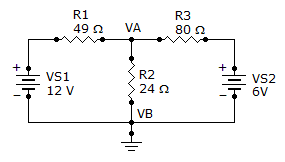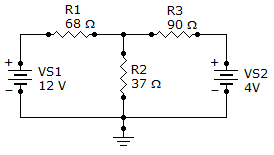# Electrical Engineering - Branch, Loop and Node Analyses

Exercise : Branch, Loop and Node Analyses - General Questions
11.
What is the current through R2?177 mA
250 mA
500 mA
125 mA
Explanation:
No answer description is available. Let's discuss.

12.
The first row of a certain determinant has the numbers 3 and 5. The second row has the numbers 7 and 2. The value of this determinant is
31
–31
39
–29
Explanation:
No answer description is available. Let's discuss.

13.
The first row of a certain determinant has the numbers 10 and 6. The second row has the numbers 3 and 5. The value of this determinant is
18
50
32
–32
Explanation:
No answer description is available. Let's discuss.

14.
What is the voltage drop across R3?112 mV
11.25 V
180 mV
1.80 V